# Vertices, Edges and Faces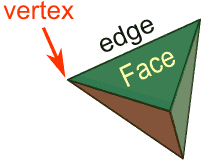A vertex is a corner.
An edge joins one vertex with another.
A face is an individual surface
.

Let us look more closely at each of those:

## Vertices

 A vertex (plural: vertices) is a point where two or more straight lines meet. It is a Corner. This tetrahedron has 4 vertices.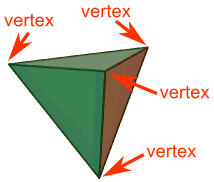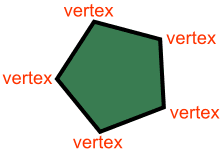And this pentagon has 5 vertices

## Edges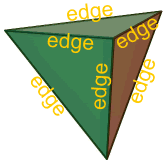An edge is a line segment that joins two vertices. This tetrahedron has 6 edges. And this pentagon has 5 edges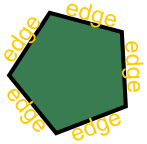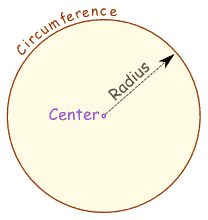It can also be the boundary of a shape. Such as the circumference of a circle.

## Faces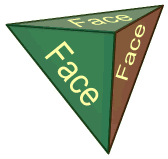A face is any of the individual surfaces of a solid object. This tetrahedron has 4 faces (there is one face you can't see)

## Euler's Formula

For many solid shapes the

• Number of Faces
• plus the Number of Vertices
• minus the Number of Edges

always equals 2

This can be written: F + V - E = 2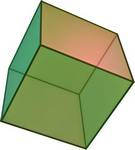Try it on the cube: A cube has 6 Faces, 8 Vertices, and 12 Edges, so: 6 + 8 - 12 = 2